# Polyphase motor design

Perhaps the most important benefit of polyphase AC power over single-phase is the design and operation of AC motors. As we studied in the first chapter of this book, some types of AC motors are virtually identical in construction to their alternator (generator) counterparts, consisting of stationary wire windings and a rotating magnet assembly. (Other AC motor designs are not quite this simple, but we will leave those details to another lesson).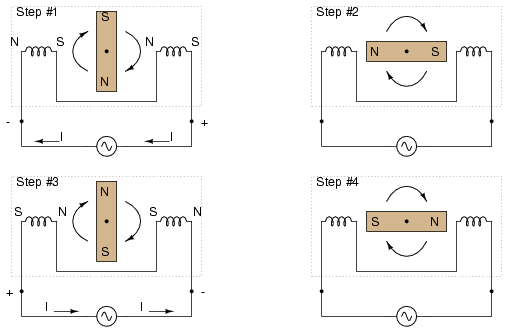Clockwise AC motor operation.

If the rotating magnet is able to keep up with the frequency of the alternating current energizing the electromagnet windings (coils), it will continue to be pulled around clockwise. (Figure However, clockwise is not the only valid direction for this motor’s shaft to spin.

It could just as easily be powered in a counter-clockwise direction by the same AC voltage waveform a in Figure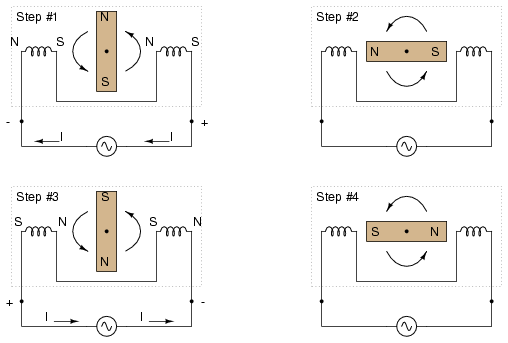Counterclockwise AC motor operation.

Notice that with the exact same sequence of polarity cycles (voltage, current, and magnetic poles produced by the coils), the magnetic rotor can spin in either direction. This is a common trait of all single-phase AC “induction” and “synchronous” motors: they have no normal or “correct” direction of rotation. The natural question should arise at this point: how can the motor get started in the intended direction if it can run either way just as well? The answer is that these motors need a little help getting started. Once helped to spin in a particular direction. they will continue to spin that way as long as AC power is maintained to the windings.

Where that “help” comes from for a single-phase AC motor to get going in one direction can vary. Usually, it comes from an additional set of windings positioned differently from the main set, and energized with an AC voltage that is out of phase with the main power. (FigureUnidirectional-starting AC two-phase motor.

These supplementary coils are typically connected in series with a capacitor to introduce a phase shift in current between the two sets of windings. (FigureCapacitor phase shift adds second phase.

That phase shift creates magnetic fields from coils 2a and 2b that are equally out of step with the fields from coils 1a and 1b. The result is a set of magnetic fields with a definite phase rotation. It is this phase rotation that pulls the rotating magnet around in a definite direction.

Polyphase AC motors require no such trickery to spin in a definite direction. Because their supply voltage waveforms alrady have a definite rotation sequence, so do the respective magnetic fields generated by the motor’s stationary windings. In fact, the combination of all three phase winding sets working together creates what is often called a rotating magnetic field. It was this concept of a rotating magnetic field that inspired Nikola Tesla to design the world’s first polyphase electrical systems (simply to make simpler, more efficient motors). The line current and safety advantages of polyphase power over single phase power were discovered later.

What can be a confusing concept is made much clearer through analogy. Have you ever seen a row of blinking light bulbs such as the kind used in Christmas decorations? Some strings appear to “move” in a definite direction as the bulbs alternately glow and darken in sequence. Other strings just blink on and off with no apparent motion. What makes the difference between the two types of bulb strings? Answer: phase shift!

Examine a string of lights where every other bulb is lit at any given time as in (Figure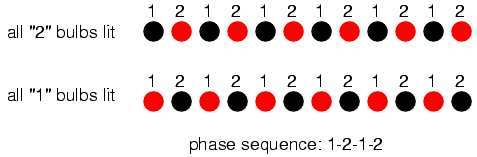Phase sequence 1-2-1-2: lamps appear to move.

When all of the “1” bulbs are lit, the “2” bulbs are dark, and vice versa. With this blinking sequence, there is no definite “motion” to the bulbs’ light. Your eyes could follow a “motion” from left to right just as easily as from right to left. Technically, the “1” and “2” bulb blinking sequences are 180o out of phase (exactly opposite each other). This is analogous to the single-phase AC motor, which can run just as easily in either direction, but which cannot start on its own because its magnetic field alternation lacks a definite “rotation.”

Now let’s examine a string of lights where there are three sets of bulbs to be sequenced instead of just two, and these three sets are equally out of phase with each other in FigurePhase sequence: 1-2-3: bulbs appear to move left to right.

If the lighting sequence is 1-2-3 (the sequence shown in (Figure ), the bulbs will appear to “move” from left to right.

Now imagine this blinking string of bulbs arranged into a circle as in Figure >.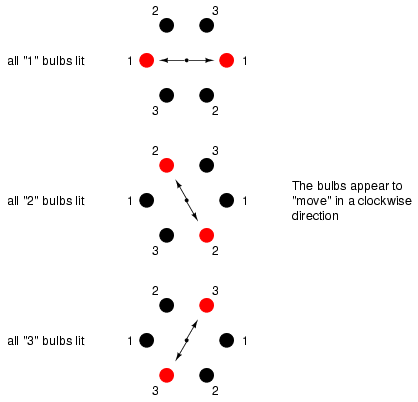Circular arrangement; bulbs appear to rotate clockwise.

Now the lights in Figure appear to be “moving” in a clockwise direction because they are arranged around a circle instead of a straight line. It should come as no surprise that the appearance of motion will reverse if the phase sequence of the bulbs is reversed.

The blinking pattern will either appear to move clockwise or counter-clockwise depending on the phase sequence. This is analogous to a three-phase AC motor with three sets of windings energized by voltage sources of three different phase shifts in Figure .Three-phase AC motor: A phase sequence of 1-2-3 spins the magnet clockwise, 3-2-1 spins the magnet counterclockwise.

With phase shifts of less than 180o we get true rotation of the magnetic field. With single-phase motors, the rotating magnetic field necessary for self-starting must to be created by way of capacitive phase shift. With polyphase motors, the necessary phase shifts are there already. Plus, the direction of shaft rotation for polyphase motors is very easily reversed: just swap any two “hot” wires going to the motor, and it will run in the opposite direction!

• REVIEW:
• AC “induction” and “synchronous” motors work by having a rotating magnet follow the alternating magnetic fields produced by stationary wire windings.
• Single-phase AC motors of this type need help to get started spinning in a particular direction.
• By introducing a phase shift f less than 180o to the magnetic fields in such a motor, a definite direction of shaft rotation can be established.
• Single-phase induction motors often use an auxiliary winding connected in series with a capacitor to create the necessary phase shift.
• Polyphase motors don’t need such measures; their direction of rotation is fixed by the phase sequence of the voltage they’re powered by.
• Swapping any two “hot” wires on a polyphase AC motor will reverse its phase sequence, thus reversing its shaft rotation.

## Three-phase Y and Δ configurations

Initially we explored the idea of three-phase power systems by connecting three voltage sources together in what is commonly known as the “Y” (or “star”) configuration. This configuration of voltage sources is characterized by a common connection point joining one side of each source. (Figure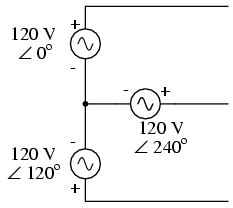Three-phase “Y” connection has three voltage sources connected to a common point.

If we draw a circuit showing each voltage source to be a coil of wire (alternator or transformer winding) and do some slight rearranging, the “Y” configuration becomes more obvious in FigureThree-phase, four-wire “Y” connection uses a “common” fourth wire.

The three conductors leading away from the voltage sources (windings) toward a load are typically called lines, while the windings themselves are typically called phases. In a Y-connected system, there may or may not (Figure ) be a neutral wire attached at the junction point in the middle, although it certainly helps alleviate potential problems should one element of a three-phase load fail open, as discussed earlier.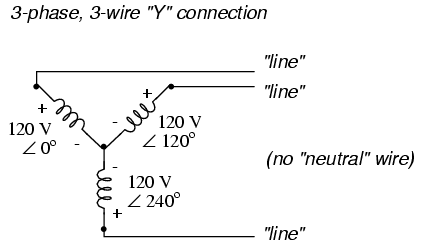Three-phase, three-wire “Y” connection does not use the neutral wire.

When we measure voltage and current in three-phase systems, we need to be specific as to where we’re measuring. Line voltage refers to the amount of voltage measured between any two line conductors in a balanced three-phase system. With the above circuit, the line voltage is roughly 208 volts. Phase voltage refers to the voltage measured across any one component (source winding or load impedance) in a balanced three-phase source or load. For the circuit shown above, the phase voltage is 120 volts. The terms line current and phase current follow the same logic: the former referring to current through any one line conductor, and the latter to current through any one component.

Y-connected sources and loads always have line voltages greater than phase voltages, and line currents equal to phase currents. If the Y-connected source or load is balanced, the line voltage will be equal to the phase voltage times the square root of 3: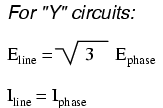However, the “Y” configuration is not the only valid one for connecting three-phase voltage source or load elements together. Another configuration is known as the “Delta,” for its geometric resemblance to the Greek letter of the same name (Δ). Take close notice of the polarity for each winding in FigureThree-phase, three-wire Δ connection has no common.

At first glance it seems as though three voltage sources like this would create a short-circuit, electrons flowing around the triangle with nothing but the internal impedance of the windings to hold them back. Due to the phase angles of these three voltage sources, however, this is not the case.

One quick check of this is to use Kirchhoff’s Voltage Law to see if the three voltages around the loop add up to zero. If they do, then there will be no voltage available to push current around and around that loop, and consequently there ill be no circulating current. Starting with the top winding and progressing counter-clockwise, our KVL expression looks something like this: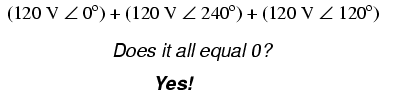Indeed, if we add these three vector quantities together, they do add up to zero. Another way to verify the fact that these three voltage sources can be connected together in a loop without resulting in circulating currents is to open up the loop at one junction point and calculate voltage across the break: (Figure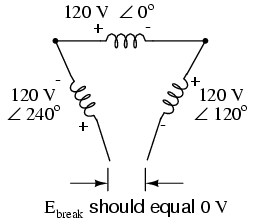Voltage across open Δ should be zero.

Starting with the right winding (120 V ∠ 120o) and progressing counter-clockwise, our KVL equation looks like this: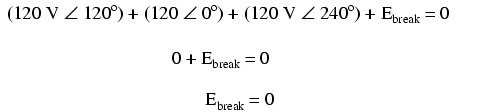Sure enough, there will be zero voltage across the break, telling us that no current will circulate within the triangular loop of windings when that connection is made complete.

Having established that a Δ-connected three-phase voltage source will not burn itself to a crisp due to circulating currents, we turn to its practical use as a source of power in three-phase circuits. Because each pair of line conductors is connected directly across a single winding in a Δ circuit, the line voltage will be equal to the phase voltage. Conversely, because each line conductor attaches at a node between two windings, the line current will be the vector sum of the two joining phase currents. Not surprisingly, the resulting equations for a Δ configuration are as follows: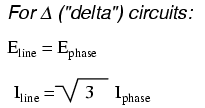Let’s see how this works in an example circuit: (Figure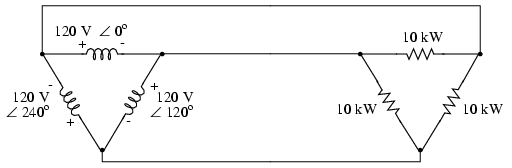The load on the Δ source is wired in a Δ.

With each load resistance receiving 120 volts from its respective phase winding at the source, the current in each phase of this circuit will be 83.33 amps: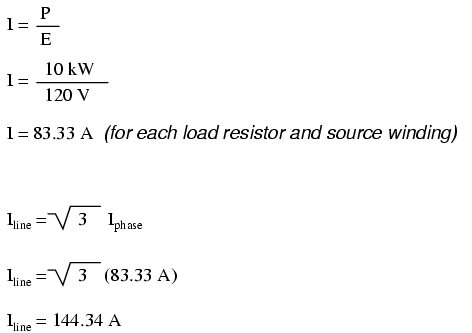So each line current in this three-phase power system is equal to 144.34 amps, which is substantially more than the line currents in the Y-connected system we looked at earlier. One might wonder if we’ve lost all the advantages of three-phase power here, given the fact that we have such greater conductor currents, necessitating thicker, more costly wire. The answer is no. Although this circuit would require three number 1 gage copper conductors (at 1000 feet of distance between source and load this equates to a little over 750 pounds of copper for the whole system), it is still less than the 1000+ pounds of copper required for a single-phase system delivering the same power (30 kW) at the same voltage (120 volts conductor-to-conductor).

One distinct advantage of a Δ-connected system is its lack of a neutral wire. With a Y-connected system, a neutral wire was needed in case one of the phase loads were to fail open (or be turned off), in order to keep the phase voltages at the load from changing. This is not necessary (or even possible!) in a Δ-connected circuit. With each load phase element directly connected across a respective source phase winding, the phase voltage will be constant regardless of open failures in the load elements.

Perhaps the greatest advantage of the Δ-connected source is its fault tolerance. It is possible for one of the windings in a Δ-connected three-phase source to fail open (Figure ) without affecting load voltage or current!Even with a source winding failure, the line voltage is still 120 V, and load phase voltage is still 120 V. The only difference is extra current in the remaining functional source windings.

The only consequence of a source winding failing open for a Δ-connected source is increased phase current in the remaining windings. Compare this fault tolerance with a Y-connected system suffering an open source winding in Figure .Open “Y” source winding haves the voltage on two loads of a Δ connected load.

With a Δ-connected load, two of the resistances suffer reduced voltage while one remains at the original line voltage, 208. A Y-connected load suffers an even worse fate (Figure ) with the same winding failure in a Y-connected sourceOpen source winding of a “Y-Y” system halves the voltage on two loads, and looses one load entirely.

In this case, two load resistances suffer reduced voltage while the third loses supply voltage completely! For this reason, Δ-connected sources are preferred for reliability. However, if dual voltages are needed (e.g. 120/208) or preferred for lower line currents, Y-connected systems are the configuration of choice.

• REVIEW:
• The conductors connected to the three points of a three-phase source or load are called lines.
• The three components comprising a three-phase source or load are called phases.
• Line voltage is the voltage measured between any two lines in a three-phase circuit.
• Phase voltage is the voltage measured across a single component in a three-phase source or load.
• Line current is the current through any one line between a three-phase source and load.
• Phase current is the current through any one component comprising a three-phase source or load.
• In balanced “Y” circuits, line voltage is equal to phase voltage times the square root of 3, while line current is equal to phase current.
•• In balanced Δ circuits, line voltage is equal to phase voltage, while line current is equal to phase current times the square root of 3.
•• Δ-connected three-phase voltage sources give greater reliability in the event of winding failure than Y-connected sources. However, Y-connected sources can deliver the same amount of power with less line current than Δ-connected sources.

Related

COMMENT songs